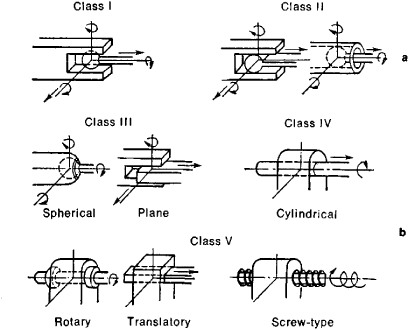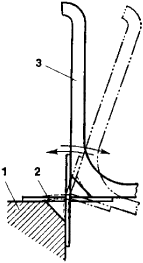# Kinematic Pair

Also found in: Wikipedia.
The following article is from The Great Soviet Encyclopedia (1979). It might be outdated or ideologically biased.

## Kinematic Pair

a movable coupling of two rigid members that imposes restraints on the relative motion of the members by the conditions of linkage. Each of the conditions of linkage removes one degree of freedom—that is, the possibility of one of the six independent relative motions in space. Three translational motions (in the direction of the three coordinate axes) and three rotational motions (around the axes) are possible in the rectangular system of coordinates.Figure 1. Kinematic pairs: (a) higher, (b) lower

Kinematic pairs are divided into five classes according to the number of conditions of linkage S imposed by the linkage. The number of degrees of freedom of a kinematic pair W = 6 — S. Within each class kinematic pairs are subdivided into types according to the relative motions of the members that remain possible. Kinematic pairs are also classified according to the type of contact between the members as lower kinematic pairs (with surface contact) and higher kinematic pairs (with line or point contact). Higher kinematic pairs are possible in all five classes and for many types; lower pairs can be only of three classes and six types (Figure 1).Figure 2. Diagram of a play-free hinge: (1) fixed part, (2) deformable elements (flat springs), (3) lever

A distinction is also made between geometrically closed and open kinematic pairs. In geometrically closed pairs, constant contact of the surfaces is assured by the shape of components (for example, in all kinematic pairs shown in Figure 1); in open pairs, a compressive force is required for closure (for example, in a cam mechanism). Some movable couplings that have several intermediate rolling bodies (such as ball bearings and roller bearings) are also conventionally considered to be kinematic pairs; this is also true of junctions with deformable intermediate components (for example, play-free hinges of devices using flat springs; Figure 2).

N. IA. NIBERG

References in periodicals archive ?
In the considered case of geared linkages, the input conditions of the type synthesis for the kinematic chain considered at least the using of one kinematic pair with DoF=2 i.e.
Manring  presented a force analysis of the piston-bore kinematic pair and a dependency for minimum fluid-film thickness.
The subject is hydromechanical processes in main kinematic pair of SWH influenced with unsettled work kinematics of hydraulic machines running gears.
(6) The last kinematic pair in the kinematic chain of all limbs must be revolute pair if the output of the moving platform has rotation movement requirement.
To reduce power losses caused by friction, there should be fluid friction mode in every kinematic pair. 
We form a system of speed vector equations [MATHEMATICAL EXPRESSION NOT REPRODUCIBLE IN ASCII] for point A, given that the point speed which coincides with a kinematic pair formed by crank 1 and the support, is equal to 0 ([V.sub.O] = 0):
Here, in terms of kinematics, this link is specified with a virtual analogue of 4th class kinematic pair .
A [K.sub.C(-2)] connection was presented in Table 4 and is based on a universal kinematic pair. Another [K.sub.C(-2)] connection is achieved by the combination of two [K.sub.B(-1)]connections built of spherical kinematic pairs (S) (Fig.
Mutual movability of items, creating a kinematic pair, is defined by number of degrees of freedom and it complies with number of autonomous simple motions (translating and rotating ones), which the both items can perform vis-a-vis.
Among kinematic pairs in the main frame of the axial-piston HMTD--piston pair is the only kinematic pair which doesn't have hydrostatic unloading of interfaced surfaces, because of complexity of its constructive realization The complexity is determined by indeterminacy of movement of the interfaced surfaces in relation to a loading force vector.
Typically, the driving kinematic pairs are either a rotation kinematic pair, or a translation kinematic pair.
This is due to variety of structural groups that can be formed with four, six or more links and a corresponding number of kinematic pairs.

Site: Follow: Share:
Open / Close